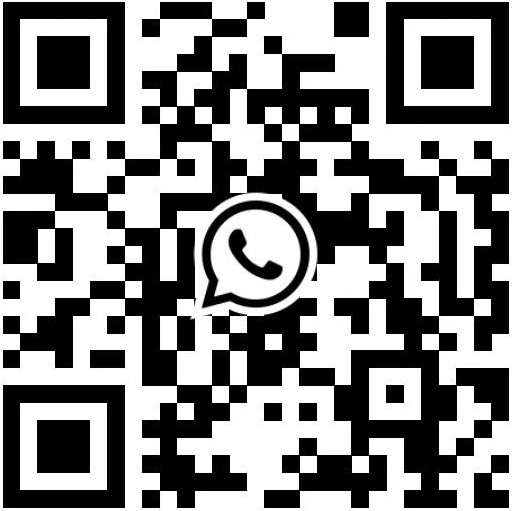# Measurement methods for laser ranging sensors

The measurement method of the laser ranging sensor is very important to the detection system, which is related to whether the detection task is successfully completed. For different detection purposes and specific situations, find a feasible measurement method, and then select a laser ranging sensor with appropriate parameters according to the measurement method. For the measurement method, starting from different angles, it can be divided into a variety of measurement methods.

According to the measurement method, it can be divided into single measurement and continuous measurement.

Single measurement One measurement order one result;

If the host does not interrupt the continuous measurement, the continuous measurement distance results up to 255 times. To interrupt a continuous measurement, the host needs to send 1 byte of 0×58 (upper case character ‘X’ in ASCII) during the measurement.

Each measurement mode has three working modes:

Automatic mode, the module returns the measurement result and signal quality (SQ), a smaller SQ value represents a more reliable distance result, in this mode the module adjusts the reading speed according to the laser reflection level;

Slow mode, higher precision;

Fast mode, higher frequency, lower precision.

According to the means of measurement, it can be divided into direct measurement and indirect measurement.

When using a sensor for measurement, the instrument reading does not require any calculations, and can directly express the results required for the measurement, which is called direct measurement. For example, after the laser distance measuring instrument directly measures, the reading is displayed on the display screen, and the measurement process is simple and fast.

Some measurands cannot or are not convenient for direct measurement, which requires calculation of the measured data to obtain the required results after using the laser distance sensor for measurement. This method is called indirect measurement.

Classified according to the change of the measured object, there are: static measurement and dynamic measurement.

The measured object is considered to be fixed during the measurement process, and this measurement is called static measurement. Static measurement does not need to consider the influence of time factors on the measurement.

If the measured object moves with the measurement process, this measurement is called dynamic measurement.

In the actual measurement process, we must start from the specific situation of the measurement task, and after careful analysis, which measurement method to use, and then decide to choose the laser distance sensor.